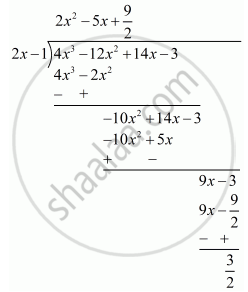Advertisement Remove all ads

# F(X) = 4x3 − 12x2 + 14x − 3, G(X) 2x − 1 - Mathematics

Answer in Brief

f(x) = 4x3 − 12x2 + 14x − 3, g(x) 2x − 1

Advertisement Remove all ads

#### Solution

Let us denote the given polynomials as

f(x) = 4x^2 - 12x^3 - 12x^2 + 14x - 3,

g(x) = 2x - 1

⇒ g (x) = 2 (x - 1/2)

We have to find the remainder when f(x) is divided by g(x).

By the remainder theorem, when f(x) is divided by g(x) the remainder is

f(1/2) = 4(1/2)^3 - 12(1/2)^2 + 14 (1/2) - 3

 = 4 xx 1/8 - 12  xx 1/4 + 14 xx 1/2 - 3

  = 1/2 -3 + 7 - 3

= 3/2

Now we will calculate the remainder by actual divisionSo the remainder by actual division is 3/2 .

Is there an error in this question or solution?
Advertisement Remove all ads

#### APPEARS IN

RD Sharma Mathematics for Class 9
Chapter 6 Factorisation of Polynomials
Exercise 6.3 | Q 4 | Page 14
Advertisement Remove all ads

#### Video TutorialsVIEW ALL 

Advertisement Remove all ads
Share
Notifications

View all notifications

Forgot password?
Course